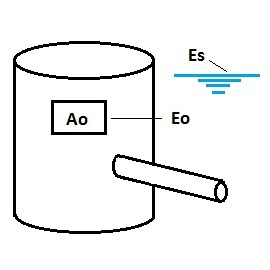* Log In to use the Calculate function * Become a Member!

Calculate Discharge Of An Orifice Outlet Control With Free Discharge:

Calculation:
Designer/Checker:

Input:(Note: All input Metric units are converted to English units for the equations below and then the output English units are converted back to Metric)

Area of orifice (Ao - SF or m^2)
Water surface elevation at design volume (Es - ft or m)
Elevation of centroid of orifice opening (Eo - ft or m)
Orifice Coeficient (C)

Typical values for C:
Orifice - sharp edge: C = 4.9
Orifice - rounded: C = 7.9
Orifice - short tube: C = 6.4
Orifice - Borda: C = 4.1

Output: